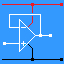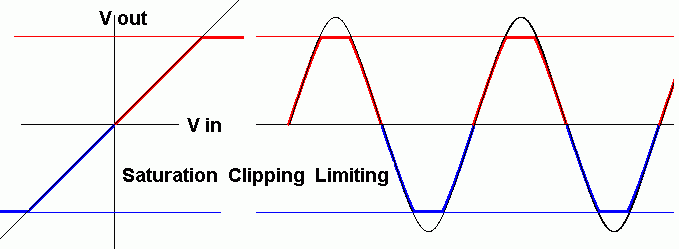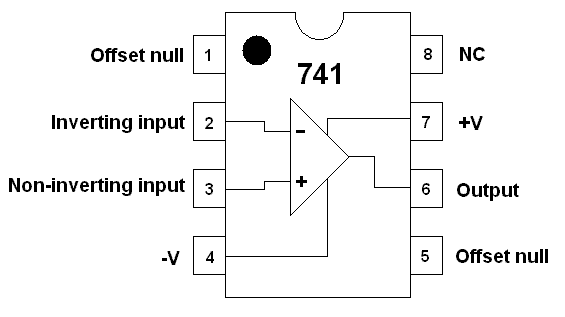RANDOM PAGE

SITE SEARCH

LOG
IN

HELP

# Op Amp Ideal Device

This is the AQA version closing after June 2019. Visit the the version for Eduqas instead.

## Ideal Operational Amplifier Properties

 Characteristics Ideal Real - Depends on the model GAIN: Open Loop Infinity > 100000 or 1000000 typical Input Resistance: (Impedance) Infinity > 1M (Bipolar) > 1000M (FET) Output Resistance: (Impedance) Zero 100 - 1000R SATURATION High: Highest Output Voltage Positive Power Supply Voltage Typical value = 12 V. Two volts below the positive supply Typical value = 10 V. SATURATION Low: Lowest Output Voltage Negative Power Supply Voltage Typical value = -12 V. Two volts above the negative supply Typical value = -10 V. Gain Bandwidth Product: Infinity 1 - 20MHz Input offset voltage: Zero Often Adjustable to Zero

## Saturation

### Clipping or Limiting

• An ideal op amp could provide an infinite output voltage range.
• A very good op amp could provide outputs at least up to the power supply voltages.
• Most op amps fall short by about two volts so with a 12 volt supply, the output would be only ten volts.
• The output should be directly proportional to the input. That is perfectly linear.

The image below shows ideal (black) and non-ideal (red and blue) behaviour including clipping when the op amp is saturated and the output voltage can go no higher.### Amplifiers increase the magnitude of the input signal.

Gain is a number (with no units) used to describe how much bigger a signal becomes when it is amplified.• Voltage Gain = Vout / Vin

• Current Gain = Currentout / Currentin

• Power Gain = Powerout / Powerin

### Amplifiers have one or more of the following properties.

• The output voltage may be bigger than the input voltage
• The output current may be bigger than the input current
• The output power may be bigger than the input power
• High input impedance (AC equivalent of resistance)
• Low output impedance (AC equivalent of resistance)

## Voltage Bandwidth

The voltage bandwidth of an op amp is the frequency over which the output is at least 70% (0.7) of the maximum output voltage of the circuit.

## Power Bandwidth

The power bandwidth of an op amp is the frequency over which the output is at least 50% (0.5) of the maximum output power of the circuit.

## The 741 Operational Amplifier

### PinoutPins

1. Offset Null - Rarely used. It can be used to to adjust for small errors in the two inputs so zero volts in gives zero volts out.
2. Inverting Input - If this voltage increases, the output voltage will go DOWN unless the Op Amp is already saturated.
3. Non-Inverting Input - If this voltage increases, the output voltage will go UP unless the Op Amp is already saturated.
4. The minus supply - Sometimes this is connected to zero volts ( ground ). Sometimes it's connected to a voltage between -5V and -18V.
5. Offset Null - Rarely used. It can be used to to adjust for small errors in the two inputs so zero volts in gives zero volts out.
6. The Output - In an ideal Op Amp, the maximum and minimum output voltage is equal to the power supply voltages. In a real life Op Amp, these voltages are about 2 to 3 Volts less.
7. The plus supply - Voltages from +5V to +18V are common. There are specialist and more expensive Op Amps with a higher voltage ratings.
8. No Connection - This pin is not used.

Data Sheet

The 741 is a low cost operational amplifier. It has ...

• A very high open loop gain (close to infinity)
• Very high input resistance (close to infinity)
• Low output resistance (close to zero)
• A rather poor gain bandwidth product compared with slightly more expensive op' amp's.
• It's very easy to use. Higher performance devices often oscillate and are much harder to work with.

It can be used to make ...

• Comparators (compare two voltages)
• Inverting Amplifiers (make the input signal bigger and invert it)
• Voltage Follower. An inverting amplifier with a voltage gain of one.
• Non Inverting Amplifiers (make the input signal bigger without inverting it)
• Summing Amplifiers (add two or more input voltages and amplify and invert the result)
• Difference (subtracting) Amplifiers (subtract two input voltages and amplify the result)
• A2 Only: Schmitt Trigger (compare the input with two different reference voltages).

reviseOmatic V3     Contacts, ©, Cookies, Data Protection and Disclaimers Hosted at linode.com, London